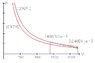# Adiabatic Process | Plotting V-P Diagram

• Karol
In summary, the gas expands from 1400 liters to 2240 liters and the pressure stays the same at 1.2 atmospheres.

## Homework Statement

A gas of volume 1400[liter] and pressure 2.33[atm] expands in an adiabatic process to 2240[liter] and 1.2[atm]. plot the process on a V-P diagram.
I shorten the question, only this interests me. the number of moles is given.

## Homework Equations

Equation of state: ##PV=nRT##

## The Attempt at a Solution

I calculated and drew the two states, see drawing. does the process go along a straight line, like the arrow, from one point to the other?

#### Attachments

•5-18.JPG
16.5 KB · Views: 441
What relates p and V in an adiabatic process? (Assume ideal gas and quasi-static process).

Now i see that i skipped the material of adiabatic process.
Pand V are related this way: $$PV^\gamma=Const$$ and the lines in the V-P diagram are curved with slopes steeper than the isotherms

Karol said:
Now i see that i skipped the material of adiabatic process.
Pand V are related this way: $$PV^\gamma=Const$$ and the lines in the V-P diagram are curved with slopes steeper than the isotherms
That is better except for the statement about the slopes of the process curves. If an isothermal and an adiabatic intersect at one point (V,p), the steepness favors one curve below that point but the other above th same point.

rude man said:
If an isothermal and an adiabatic intersect at one point (V,p), the steepness favors one curve below that point but the other above th same point.
I didn't understand that, maybe i don't know enough english by saying "favors". the slope of the adiabatic lines is always bigger than the isotherms, so which point do you mean above and below the intersection? i am not at home now and i don't have a drawing program but later i will sketch and explain my question.

Karol said:
I didn't understand that, maybe i don't know enough english by saying "favors". the slope of the adiabatic lines is always bigger than the isotherms, so which point do you mean above and below the intersection? i am not at home now and i don't have a drawing program but later i will sketch and explain my question.
Looks like you were right. Here are curves from my textbook showing an isotherm and an adiabatic curve intersecting at a point. The curves diverge as shown but you are right, the slope of the adiabatic curve is always larger in magnitude than the isotherm's. Hope I helped you with the rest anyway!PS I wanted to add the attached Hope it's legible.

#### Attachments

Last edited:
Which book is that? what's it's name and author

Karol said:
Which book is that? what's its name and author
Resnick and Halliday, Physics
It's a pretty standard introductory 2-volume set (also issued in 1 volume).
The above is the 1st volume & covers up to & incl. heat.
2nd volume is Halliday & Resnick, Physics, & covers e-m, light, & touches on wave mechanics.
Excellent texts. I tyhrew out my own textbook when I ran across these.

Thanks